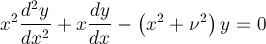Computes modified cylindrical Bessel functions.

Namespace:  Meta.Numerics.Functions
Assembly:  Meta.Numerics (in Meta.Numerics.dll) Version: 4.0.5.0 (4.0.5.0)Syntax
```public static SolutionPair ModifiedBessel(
double nu,
double x
)```

#### Parameters

nu
Type: SystemDouble
The order, which must be non-negative.
x
Type: SystemDouble
The argument, which must be non-negative.

#### Return Value

Type: SolutionPair
The values of I, I', K, and K' for the given order and argument.Remarks

The modified Bessel functions fulfill a differential equation similar to the Bessel differential equation.See Also